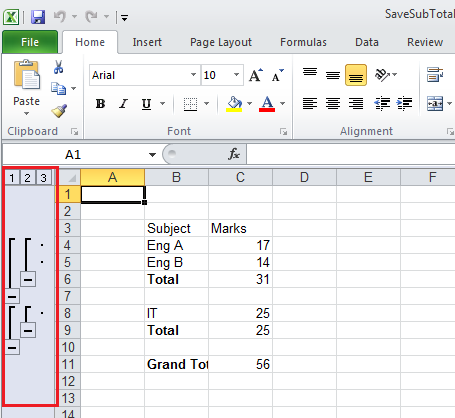In this blog, two recently added features are being discussed :

1. Subtotals

# SubTotals

In C1Excel, we can create subtotals for rows and column and also set the outline level of row/column containing the Total. This can be achieved by using Formula, OutlineLevel property. Image below shows the Excel file that has Formula, OutlineLevel applied :For example : The below line will set the outline level of the row so that a Expand ( ) or Collapse (-) sign is displayed before the row

`````` sheet.Rows.OutlineLevel = 2;
``````

Also, code line below can be used to set the formula for calculating subtotal :

``````sheet[5, 2].Formula = “SUBTOTAL(9,C4:C5)”;
``````

Following code can be used for creation of excel file with subtotals :

``````    XLStyle totalStyle = new XLStyle(book);
totalStyle.Font = new Font("Arial", 12, FontStyle.Bold) ;

XLSheet sheet = book.Sheets;
sheet[2, 1].Value = "Subject";
sheet[2, 2].Value = "Marks";
sheet[3, 1].Value = "Eng A";
sheet[3, 2].Value = 17;

//Set the Outline Level of the row
sheet.Rows.OutlineLevel = 2;
sheet.Rows.Visible = true;

sheet[4, 1].Value = "Eng B";
sheet[4, 2].Value = 14;

sheet.Rows.OutlineLevel = 2;
sheet.Rows.Visible = true;

sheet[5, 1].Value = "Total";
sheet[5, 1].Style = totalStyle;
sheet[5, 2].Value = 31;

//Used to calculate subtotal
sheet[5, 2].Formula = "SUBTOTAL(9,C4:C5)";
sheet.Rows.OutlineLevel = 1;

sheet[7, 1].Value = "IT";
sheet[7, 2].Value = 25;
sheet.Rows.OutlineLevel = 2;

sheet[8, 1].Value = "Total";
sheet[8, 1].Style = totalStyle;
sheet[8, 2].Value = 25;

sheet[8, 2].Formula = "SUBTOTAL(9,C8:C8)";
sheet.Rows.OutlineLevel = 1;

sheet[10, 1].Value = "Grand Total";
sheet[10, 1].Style = totalStyle;

sheet[10, 2].Value = 140;
sheet[10, 2].Formula = "SUBTOTAL(9,C4:C8)";

sheet.Rows.OutlineLevel = 0;

book.Save("SaveSubTotal.xlsx");   // or “Save.xls”
``````

``````c1XLBook1.Load(path, false);
``````

This event is used to load the sheet names only(not the data in sheets). Second parameter (false),means that we need to just load the sheet names and not the data in sheets. Next we will load the specific sheet using C1XLBook.Sheets[index].Load(path, bool). Following code specifies the sheet that needs to be loaded, In the above code we are loading only second sheet:

``````c1XLBook1.Sheets.Load(path, true);
``````

Thus, following code will load only first sheet of the excel file :

``````// path of file for loading (xls or xlsx)
string path = "..//..//MultipleSheets.xlsx";

// load workbook without worksheet data